# 1st PUC Computer Science Previous Year Question Paper March 2016 (North)

Students can Download 1st PUC Computer Science Previous Year Question Paper March 2016 (North), Karnataka 1st PUC Computer Science Model Question Papers with Answers helps you to revise the complete Karnataka State Board Syllabus and score more marks in your examinations.

## Karnataka 1st PUC Computer Science Previous Year Question Paper March 2016 (North)

Time: 3:15 Hours
Max. Marks: 100

PART – A

I. Answer all the following questions. Each question carries one mark. (10 × 1 = 10)

Question 1.
Name the basic electronic component used in second-generation computers.
The main electronic component of second-generation computer systems was a transistor.

Question 2.
Expand DVD.
Digital Versatile Disk

Question 3.
What is program documentation?
It is reference material that contains details about a program and functions of different programs of a software. Program Documentation:
It is a reference material that contains details about a program and functions of different programs of software. Documentation helps other users to understand the program and use it conveniently more effectively.

Question 4.
What is constant?
The program elements, which not change during the execution of a program, are known as constants.Question 5.
Give the usage of end1 function.
A manipulator is a C++ is used to control the formatting of output and/or input values. Manipulators can only be present in input/output statements. The end1 manipulator causes a newline character to be output end1 is defined in the header file and can be used as long as the header file has been included.

Question 6.
What is nested -if statement?
An if statement nestling inside another ‘if’ statement is termed as ‘a nested if’ statement.

Question 7.
Write the declaration syntax of one dimensional array.
datatype array name [size];

Question 8.
What are formal parameters?
Formal arguments are the names of the arguments/parameters in the function header of called function.Question 9.
What is header in word processor?
A header is a special area a|the top of every page normally allocated in the top margin area.

Question 10.
Mention any one type of chart in ESS.
The different categories of charts are column, line, pie, bar, xy scattered, etc.,

PART – B

II. Answer any. Five of the following questions. Each question carries two marks: (5 × 2 = 10)

Question 11.
Write a note on main frame computers.
Main frame computers are fastest and largest expensive computers. They are normally used for business computations.

Question 12.
Compare static and dynamic RAM.
Static RAM:

• SRAM uses six transistors for each memory cell.
• SRAM doesn’t need a refresher circuit.
• SRAM is costlier and used for the smaller and faster cache memory.

Dynamic RAM:

• DRAM uses a single capacitor and a transistor for each memory cell.
• DRAM requires to be refreshed periodically.
• DRAM is cheaper and slower and is used for the large main memory.

Question 13.
What is translator? Name any one translator.
The translator translates user written high level language programs to equivalent machine language programs. For example, compilers.Question 14.
What are syntax and semantic errors?
1. syntax error:
It refers to an error in the syntax of a sequence of characters or tokens that is intended to be written in a particular programming language.

2. semantic error:
An error, which occurs due to the incorrect logic and a solution is called a semantic error.

Question 15.
Give any two advantages of OOPs.
Some of the advantages of object-oriented programming are as follows:

1. A clear modular structure can be obtained which can be used as a prototype and it will not reveal the details of the design.
2. Easy maintenance and modifications to the existing objects can be done with ease.
3. A good framework is provided which facilitates creating rich GUI applications.Question 16.
What is a derived data type? Give an example.
The derived data type or user defined data type is created by using basic data types. For example, structure

Question 17.
Mention the use of isalpha() and toupper() character functions.
1. isalpha():
Returns a non-zero value if the argument is a A-Z or a-z letters.
For example, isalpha(‘a’) output: 1 means true isalpha(‘5’) output: 0 means false

2. toupper( ):
It converts the lower case alphabet character to upper case.
For example, toupper(‘a’) output: A

Question 18.
Write the shortcut keys for cut and paste operation in MS Word.
The sequence of short cut keys to cut and paste is Ctrl+X for cut and Ctrl+V for paste.

PART – C

III. Answer any Five of the following Questions. Each question carries three marks: (5 × 3 = 15)

Question 19.
What are impact printers? Briefly explain any one impact printer.
Impact printers form characters or images by striking at a mechanism such as a print hammer or wheel against an inked ribbon, leaving an image on the paper.

Question 20.
Convert the following: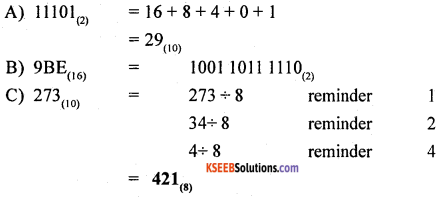Question 21.
Write the features of UNIX operating system.
The features of the UNIX operating system are:

More than one program can be run at a time

2. Portability:
Only the kernel (<10%) is written in assembler tools for program development a wide range of support tools (debuggers, compilers). The system is written in high-level language making it easier to read, understand, changed and, therefore move to other machines.

3. Machine-independence:
The System hides the machine architecture from the user, making it easier to write applications that can run on micros, mins, and mainframes.

4. Multi-User Operations:
UNIX is a multi-user system designed to support a group of users simultaneously. The system allows for the sharing of processing power and peripheral resources, while at the same time providing excellent security features.

5. Hierarchical File System:
UNIX uses a hierarchical file structure to store information. This structure has the maximum flexibility in grouping information in a way that reflectsits natural state. It allows for easy maintenance and efficient implementation.Question 22.
What is looping? Write the syntax and example for while loop construct.
A ‘while’ loop statement repeatedly executes a statement or sequence of statements written in the flower brackets as long as a given condition returns the value ‘true’.
Syntax:
The syntax of a ‘while’ loop in C++ is:
while(condition)
{
statement (s) ;
}
Here the condition may be any expression, and for true is any non zero value. The loop iterates while the condition is true. When the condition becomes false, program control passes to the line immediately following the loop. During the first attempt, when the condition is tested and the result is false, the loop body will be skipped and the first statement after the ‘while’ loop will be executed.
Example: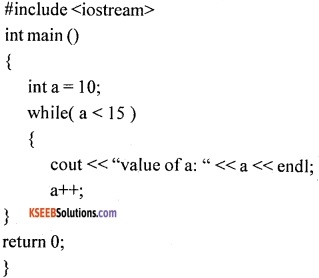When the above code is executed, it produces the following result:
value of a: 10
value of a: 11
value of a: 12
value of a: 13
value of a: 14

Question 23.
Explain any three mathematical functions in C++.
1. a cos() function:
It is a mathematical function that returns the arc cosine value for the given argument. The argument value must be in the range -1 to 1, otherwise, a domain error is triggered.
Syntax: float acos ( float arg);

2. cos() function:
It is a mathematical function that returns the cosine Value for the given radians.
Syntax: float cos( float arg );

3. ceil() function:
It is a mathematical function that returns the smallest integer value that is not less than the specified number. In other words, it returns the Round-Up value of a given number.
Syntax: float ceil( float num);

4. exp() function:
It is a mathematical function that returns the natural logarithm base “e” raised to the power equal to the argument.
Syntax: float exp( float num);

5. sqrt() function:
It is a mathematical function that returns the square root of the given “num” value.
Syntax: float sqrt( float num);Question 24.
What is the use of cin function? Write its syntax and example.
1. Input Operator:
The statement cin>> num;
is an input statement and causes the program to wait for the user to type in a number. The operator >>is known as extraction or get from the operator. It takes the value from the keyboard and assigns it to the variable on its right.

2. Output Operator:
The statement cout<< “ the numbers”;uses the cout identifier that represents the standard output stream ( screen) in C++. The operator < – Sounds incomplete -Author.

Question 25.
What is array? Write a program segment to read and print the elements of one
dimensional array.
Array is a group of similar elements that share a common name.
Using ‘for’ loop:
int age , i;
for(i=0; i<10; i++) { cin>>age[i];
}
for(i=0;i<10;i++)
cout<<age[i]; Question 26. Which operator is used to access the elements of a structure? Write its syntax and example. Answer: The accessing of data of the members is done by using the following format: structure variable.member name for example cin>>s1.rollno>>s1.age>>s1.name>>s1.marks;

PART – D

IV. Answer any Seven of the following questions. Each question carries Five marks: (7 × 5 = 35)

Question 27.
Discuss the characteristics of a computer.
1. Speed:
Computers are fast in doing calculations. The speed of the computer is measured in terms of No. of million instructions per second (MIPS).

2. Storage Capacity:
Computers come with a very large amount of memory space. They can hold a lot of data. Computers can extract a particular piece of information from a large amount of available data within a very short time.

3. Diligence:
After doing work for some time, humans become tired but computers do not become tired. They work continuously. In fact, Computers which control telephone exchanges, are never shut down. This is called diligence.

4. Accuracy:
The results that the computers produce are accurately provided that the input data and programs are reliable.

Question 28.
How do you find the 1’s complement of a number? Subtract 14(10) – 25(10) using l’s complement.
The 1’s complement of a number is formed by changing all 1’s to 0’s and all 0’s to 1’s.

Now find 1’s complement of 10100 which is 01011(2) = -11(10)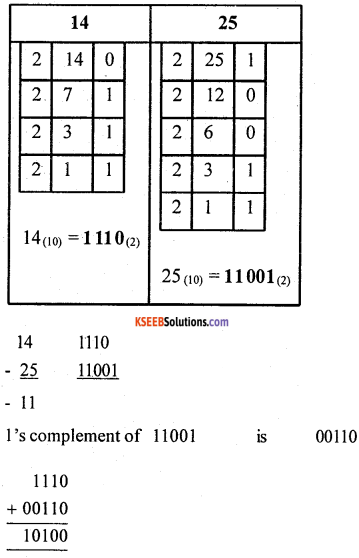Question 29.
Write a flow chart to generate the Fibonacci series up to the given limit.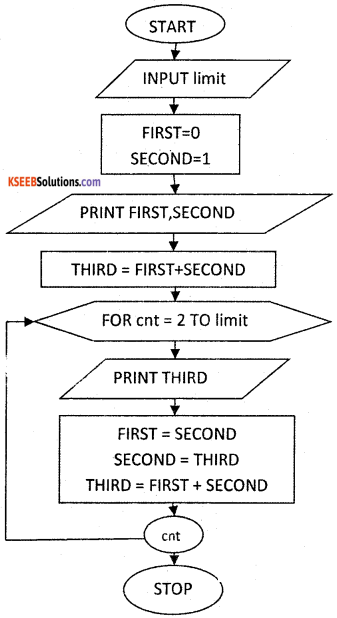Question 30.
What are identifiers? Write the rules of naming identifiers in C++.
The identifier is a sequence of characters taken from the C++ character set. The rules for the formation of an identifier are:

• It can consist of alphabets, digits and/or underscores.
• C++ is case sensitive, that is upper and lower case letters are considered as different from each other.
• It should not be a reserved keyword.

Question 31.
Explain switch statement with a suitable example in C++.
‘ Switch’ statement compares the value of an expression against a list of integers or character constants. The list of constants are listed using the “case” statement along with a “break” statement to end the execution.
Example: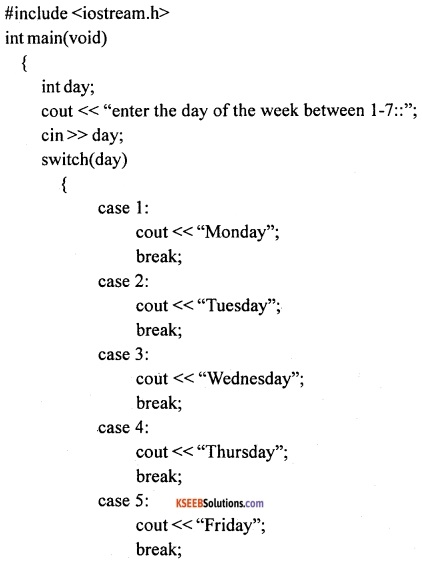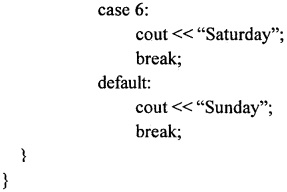Result:
Enter the day of the week between 1-7::7
Sunday

In the above Control Structure example, the “switch” statement is used to find the day of the week from the integer input got from the user. The value present in the day is compared for equality with constants written in the word case. Since no equality is achieved in the above example (from 1 to 6), when 7 is entered default is selected and gives “Sunday” as a result.Question 32.
Write an algorithm to check the given number is a power of 2.

• Step 1: start
• Step 2: input n
• Step 3: let temp = n
• Step 4: let status =1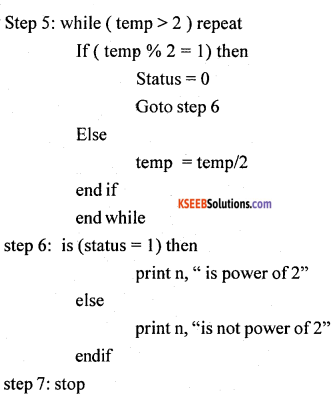Question 33.
How do you access the elements of a two dimensional array? Write the syntax and example for two dimensional array initialization.
The row index and column index number of two dimensional array along with array name is “r” used to access the elements of a two dimensional array.

Question 34.
What is recursive function? Give the syntax and example for it.
1. A function that calls itself directly or indirectly, again and again, is called recursive functions and the process is termed as recursion.

2. A function is called ‘recursive’ if a statement within the body of that function calls the same function.

3. The most common example of a recursive function is the calculation of the factorial of a number. i.e., n! = (n) * (n-1)

In the above formula, the second part (n-1)! is calling the formula again.
Program: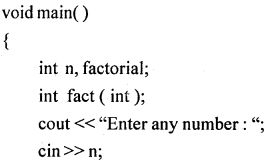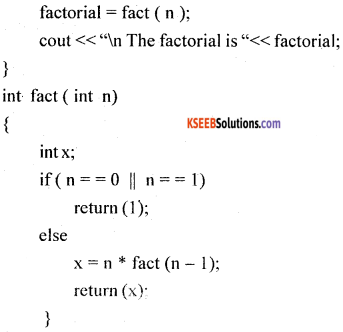In the above example, the calling function main () gives the function call to fact () and co, To jumps from main () function to called function fact(). The argument ‘n’ value is compared with the base class ‘if (n == 1)’ if ‘true’ control will return back to calling function main().

If ‘False’, control will execute the statement which is after ‘else’ x = n * fact ( n – 1);. here a function call is given to fact (n-1) with the parameter (n-1). Now the calling function is fact() and called function is also fact( ). The recursion ends when value of ‘n’ is 1 then the statement return (1) is initiated.Question 35.
What is a spread sheet? Explain any four formatting options in ESS.
A spread sheet is a document which is divided into rows and columns to store data such as items of an inventory, income, and expenses, salary statement, etc.

Question 36.
Explain any five statistical functions in ESS.
1. = average() is a statistical functions that computes average of a series.
2. = max() function is used to find the maximum value of a series.
3. = min() function is used the find the minimum value of a series.
4. = count () function is used the return the number of values present in a series.
5. = stdev() function estimates the standard deviation based on a sample.

Question 37.
Explain the different internet services.
The services of internet are email, voice mail chat, Bullet inboard, newsgroup, FTP, WWW, etc.,

1. email:
It is a system for exchanging written, voice and video messages through a computer network. Some uses of email are as follows;

• Messages can be sent 24 × 7 at any convenient time that suits the user.
• Messages reach the recipient’s mailbox within minutes.
• Delivery of the messages can be confirmed.

2. Voice Mail:
Voice mail messages are stored on hard disk drives, a media generally used by computers. Messages are recorded in digitized from the natural human voice. To retrieve messages, a user calls the system from any phone, logs on, and their messages can be retrieved immediately.

3. Chat:
It is an online communication between two users, through a computer on the internet. Once a chat has been initiated, either user can enter text by typing on the keyboard and the entered text will appear on the other user’s monitor. Most networks and online services offer a chat facility.

4. Bulletin Board:
It is an electronic message center where people can post public messages, messages are placed on the computer so people can leave them for other people to read and see and erase when the need is oyen it is used, For example, to advertise things to buy or sell, announce events, or provide information.

5. FTP:
It is a standard network protocol used to exchange and manipulate files over a TCP/ IP based network, such as the Internet. FTP is most commonly used to download a file from a server using the Internet or to upload a file to a server.

6. Telnet:
Telnet stands for ‘telecommunications network’, and was built to be a form of remote control to manage mainframe computers from distant terminals. Telnet is a text-only protocol, and to user cannot see graphics or fancy images while working with Telnet, nor will they be able to transfer files with Telnet. Telnet is used to communicate with other computers and machines in a text-based manner.